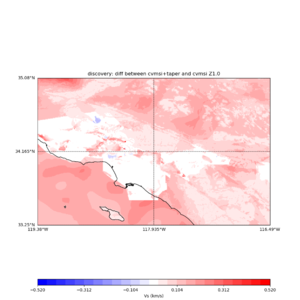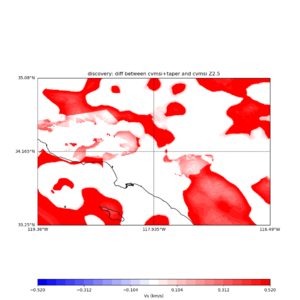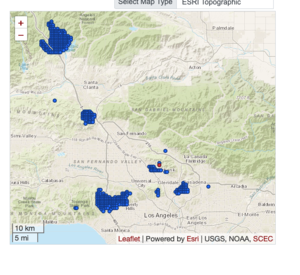# UCVM cvmsi tapering for CyberShake Study 22.12

## UCVM tapering

interp Zrange is set to [0,700] initially

related ucvm_query flags that affects tapering:

-L vs,vp,density (optional)

```     example,  -L 200,-1,-1
(only floor the vs to 200)
```

-m cvmsi,elygtl:taper

```         (adding tapering interp to cvmsi)
```

-z zmin,zmax (optional)

```         (zrange values, original default: zmin = 0, zmax = 350)
```

UCVM's implementation of ucvm-tapering is ..

```     zmax fixed at 700

outside of zrange

below zmin,    process GTL (vs30 to vs)
above zmax,    straight CRUST pass thru

inside of range

calculate target-taper values

*** THIS IS NEW ***
to get the original interp_crust value
and brought it back from backend to use for this
--- only implemented for plugin modules
*** What does this mean?
unless the non-plugin module set these values,
tapering is not going to work.  ie. cvmh
can not be tapered, (not sure if it makes sense)
but cvmhlabn could be tapered.

if interp_crust.vs < target-taper.vs
use interp_crust
else
use target-taper

default vs_floor 500, vp_floor 1700, density_floor 1700
if there is a user-defined floor from ucvm_query command line option, use user-defined floors

orig_ratio= vp/vs

Rule 1,
if vs < vs_floor:
vs=vs_floor
vp=vs_floor * orig_ratio

Rule 2,
if vp < vp_floor:
vp=vp_floor
Rule 3,
if density < density_floor:
density=density_floor

Rule 4,
if (vp/vs) < 1.45:
vs=vp/1.45

```
```#define UCVM_DEFAULT_VS_FLOOR 500.0
#define UCVM_DEFAULT_VP_FLOOR 1700.0
#define UCVM_DEFAULT_DENSITY_FLOOR 1700.0
```

## Generate Z1.0 and Z2.5

on Discovery, commands used to create Z1.0 and Z2.5 basin query results,

```#!/bin/bash
if [ -z "\$UCVM_INSTALL_PATH" ]; then
echo "Need to set UCVM_INSTALL_PATH to run >" \${0##*/}
exit
fi
source \$UCVM_INSTALL_PATH/conf/ucvm_env.sh

BIN_DIR=\${UCVM_INSTALL_PATH}/bin
CONF_DIR=\${UCVM_INSTALL_PATH}/conf

TEST=basin_query_mpi_complete_cvmsi_taper_z2.5
salloc \${UCVM_SALLOC_ENV} -Q --nodes=2 --ntasks=4 --mem=16GB --time=01:00:00 srun -Q -o \${TEST}.srun.out \${BIN_DIR}/basin_query_mpi_complete -b \${TES
T}.first,\${TEST}.firstOrSecond,\${TEST}.last,\${TEST}.secondOnly,\${TEST}.threeLast -o \${TEST}.result,\${TEST}.meta.json -f \${CONF_DIR}/ucvm.conf -m cvms
i,elygtl:taper -i 10 -v 2500 -l 33.25,-119.38 -s 0.005 -x 578 -y 366

TEST=basin_query_mpi_complete_cvmsi_taper_z1.0
salloc \${UCVM_SALLOC_ENV} -Q --nodes=2 --ntasks=4 --mem=16GB --time=01:00:00 srun -Q -o \${TEST}.srun.out \${BIN_DIR}/basin_query_mpi_complete -b \${TES
T}.first,\${TEST}.firstOrSecond,\${TEST}.last,\${TEST}.secondOnly,\${TEST}.threeLast -o \${TEST}.result,\${TEST}.meta.json -f \${CONF_DIR}/ucvm.conf -m cvms
i,elygtl:taper -i 10 -v 1000 -l 33.25,-119.38 -s 0.005 -x 578 -y 366
```

the .firstOrSecond files are the ones that is needed for CyberShake study

## Plot the binary file

To plot these files with ucvm_plotting, data ingest mode of plot_z10_map.py and plot_z25_map.py from ucvm_plotting is used but first needs to change those file name to have .binary file name so plotting scripts know how to parse the data

```plot_z10_map.py -a s -s 0.0050 -b 33.25,-119.38 -u 35.08,-116.49 -c cvmsi,elygtl:taper -x 578 -y 366 -f cvmsi_taper_z1.0.binary -o cvmsi_taper_z1.0.png
plot_z25_map.py -a s -s 0.005 -b 33.25,-119.38 -u 35.08,-116.49 -c cvmsi,elygtl:taper -x 578 -y 366 -f cvmsi_taper_z2.5.binary -o cvmsi_taper_z2.5.png

plot_z10_map.py -a s -s 0.0050 -b 33.25,-119.38 -u 35.08,-116.49 -c cvmsi -x 578 -y 366 -f cvmsi_z1.0.binary -o cvmsi_z1.0.png
plot_z25_map.py -a s -s 0.005 -b 33.25,-119.38 -u 35.08,-116.49 -c cvmsi -x 578 -y 366 -f cvmsi_z2.5.binary -o cvmsi_z2.5.png
```

An example of the output,

```Using parameters:

datafile = cvmsi_taper_z1.0.binary
lat1 = 33.25
lat2 = 35.08
color = s
outfile = cvmsi_taper_z1.0.png
spacing = 0.0050
nx = 578
ny = 366
cvm = cvmsi,elygtl:taper
lon1 = -119.38
lon2 = -116.49

TOTAL number of binary data read:211548
```

## Create difference map between 2 binary files

```plot_vs30_etree_difference_map.py -s 0.005 -c cvmsi -a d -i \${UCVM_INSTALL_PATH} -b 33.25,-119.38 -u 35.08,-116.49 -o diff_taper_none_z1.0.png -f cvmsi_taper_z1.0.binary,cvmsi_z1.0.binary -x 578 -y 366 -t "diff between cvmsi and cvmsi+taper Z1.0" -A -0.52,0.52

plot_vs30_etree_difference_map.py -s 0.005 -c cvmsi -a d -i \${UCVM_INSTALL_PATH} -b 33.25,-119.38 -u 35.08,-116.49 -o diff_taper_none_z2.5.png -f cvmsi_taper_z2.5.binary,cvmsi_z2.5.binary -x 578 -y 366 -t "diff between cvmsi and cvmsi+taper Z2.5" -A -0.52,0.52
```

An example of the output :

```Using parameters:

ny = 366
lat1 = 33.25
datafile2 = cvmsi_z1.0.binary
datafile1 = cvmsi_taper_z1.0.binary
lat2 = 35.08
color = d
title = diff between cvmsi and cvmsi+taper Z1.0
spacing = 0.005
nx = 578
outfile = diff_taper_none_z1.0.png
cvm = cvmsi
lon1 = -119.38
scalemax = 0.52
scalemin = -0.52
lon2 = -116.49
installdir = /var/www/html/UCVM_web/web/model/UCVM_TARGET

Using --> cvmsi_taper_z1.0.binary
TOTAL number of binary data read:211548

Using --> cvmsi_z1.0.binary
TOTAL number of binary data read:211548
```difference map, CVMSI, taper/none, z1.0difference map, CVMSI, taper/none, z2.5

## What is actual Z1.0 and Z2.5 for a point

LAPD point -118.125 34.557

```#!/bin/sh

echo "z2.5 with taper"
basin_query -m cvmsi,elygtl:taper -v 2500.0 < in

echo "z2.5 no taper"
basin_query -m cvmsi -v 2500.0 < in

echo "z1.0 with taper"
basin_query -m cvmsi,elygtl:taper -v 1000.0 < in

echo "1.0 no taper"
basin_query -m cvmsi -v 1000.0 < in

```
```
using LAPD z2.5 with taper
-118.1250    34.5570    580.000    580.000    580.000
z2.5 no taper
-118.1250    34.5570      0.000      0.000      0.000
z1.0 with taper
-118.1250    34.5570    120.000    120.000    120.000
1.0 no taper
-118.1250    34.5570      0.000      0.000      0.000

```

## Blue mystery spots

A study of Z1.0 loc where the difference range from 0 to -270. There are 441 spotsdifference map, CVMSI, taper/none, z1.0

Data file,